Question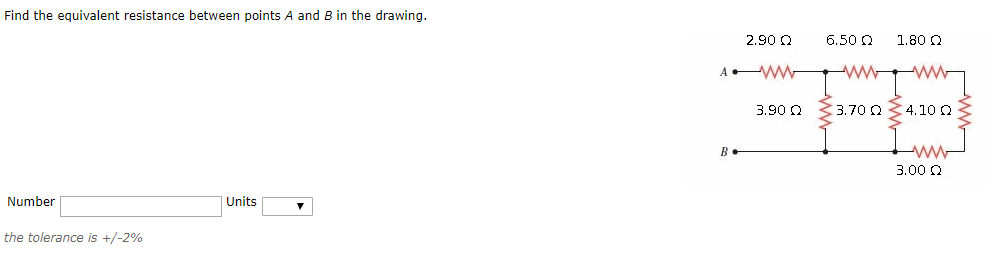Solution)

Here, 1.8 ohms, 4.1 ohms and 3 ohms are in series

So, Req= 1.8+4.1+3= 8.9 ohms

Now, the combination of 8.9 ohms is in parallel with 3.7 ohms

So, Req= 8.9*3.7/(8.9+3.7) = 2.61 ohms

Now, 2.6 ohms in series with 6.5 ohms

So, Req= 2.6 + 6.5= 9.11 ohms

Now, 9.11 ohms in parallel with 3.9 ohms

So, Req= 9.11*3.9/(9.11+3.9)= 2.73 ohms

Now, 2.73 ohms in series with 2.9 ohms

So, Req= 2.73+2.9 = 5.63 ohms (Ans)

=========

Good luck!:)

#### Earn Coins

Coins can be redeemed for fabulous gifts.

Similar Homework Help Questions
• ### Find the equivalent resistance between points A and B in the drawing. Find the equivalent resistance...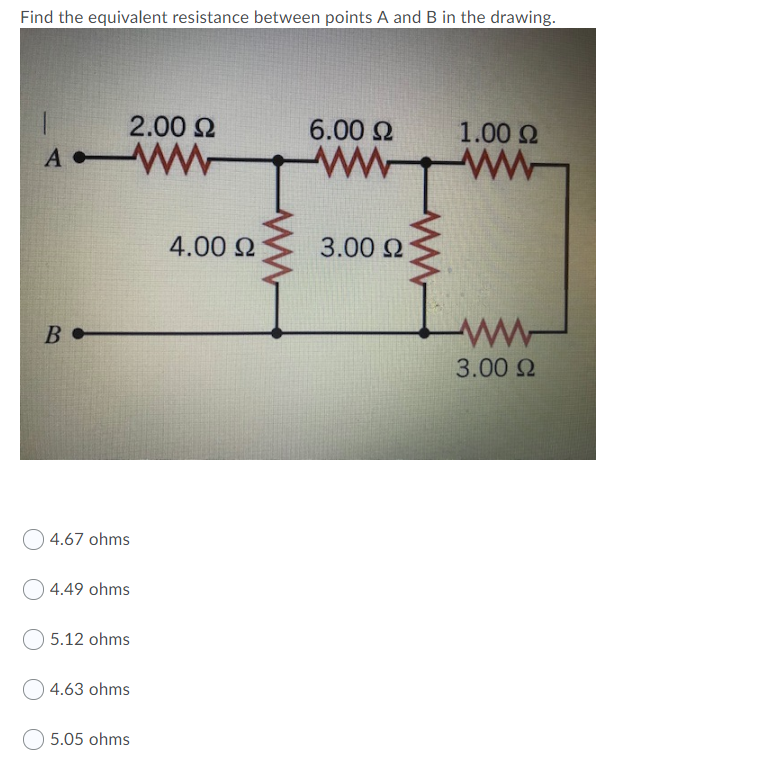Find the equivalent resistance between points A and B in the drawing. Find the equivalent resistance between points A and B in the drawing. 2.00 Ω 6.00 Ω 1.00 Ω A 4.00 Ω 3.00 Ω B - ΑΛΛ- 3.00 Ω 4.67 ohms Ο4.49 ohms 5.12 ohms 4.63 ohms 5.05 ohms

• ### Find the equivalent resistance between points A and B in the drawing 2.00 Ω 6.00 Ω...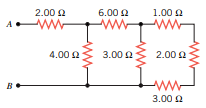Find the equivalent resistance between points A and B in the drawing 2.00 Ω 6.00 Ω 1.00 Ω wongan 4.00 Ω 3.00Ω 2.00 Ω B 3.00 Ω

• ### Find the equivalent resistance between points A and B in the drawing. 3.0 Ω 4.0 2...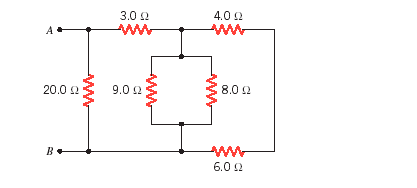Find the equivalent resistance between points A and B in the drawing. 3.0 Ω 4.0 2 8.0 Ω 20012 9.0 12 6.0 Ω

• ### Find the equivalent resistance between points A and B in the drawing. RI 26 Ω R2...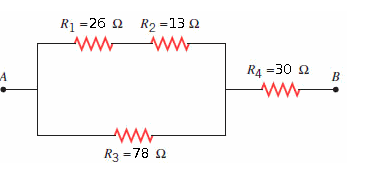Find the equivalent resistance between points A and B in the drawing. RI 26 Ω R2 1362 R4-30 Ω Ra 78 Ω

• ### 4. Find the equivalent resistance between points A and B in the drawing (R1 = 4.0...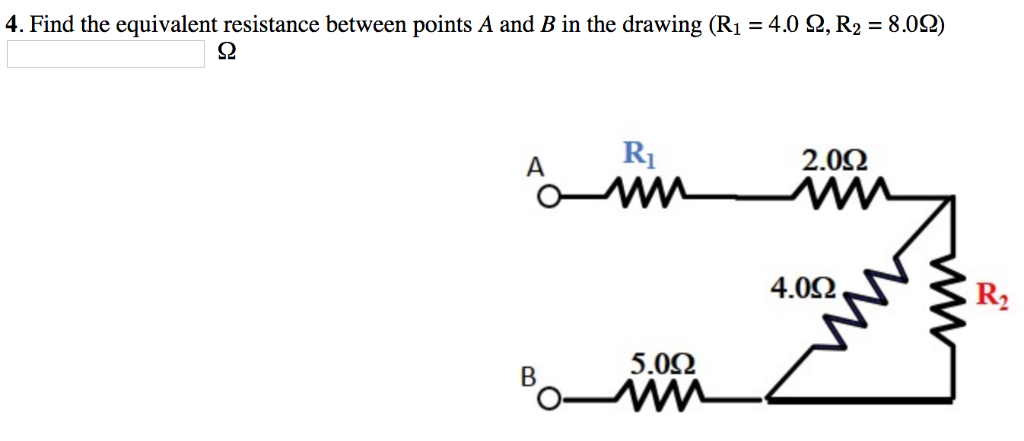4. Find the equivalent resistance between points A and B in the drawing (R1 = 4.0 Ω, R2 = 8.0Ω) S2 2.0Ω 4.0Ω R2 5.0Ω

• ### Find the equivalent resistance between points A and B in the drawing. Number____ Units____

Find the equivalent resistance between points A and B in the drawing. Number____ Units____

• ### Find the equivalent resistance between points A and B in the drawing. 3.0 4.0 A, a...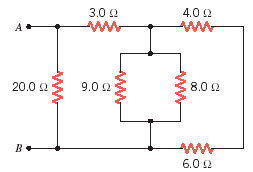Find the equivalent resistance between points A and B in the drawing. 3.0 4.0 A, a WW WW 20.0 9.0 2 8.0 6.0

• ### The equivalent resistance between points A and B of the circuit shown is 25.2 Ω. For...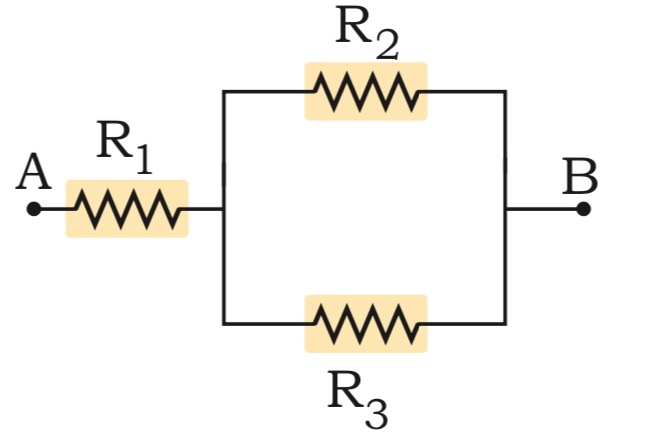The equivalent resistance between points A and B of the circuit shown is 25.2 Ω. For R1 = 10.3 Ω and R2 = 52.8 Ω, calculate the value of resistance R3. A B 1

• ### (a) Find the equivalent resistance between points a and b in Figure P18.2. (b) Calculate the...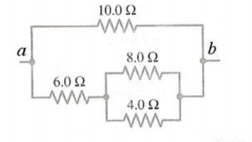(a) Find the equivalent resistance between points a and b in Figure P18.2. (b) Calculate the current in each resistor if a potential difference of 34.0 V is applied between points a and b. 10.0 Ω 8.0 Ω 4.0 Ω